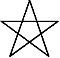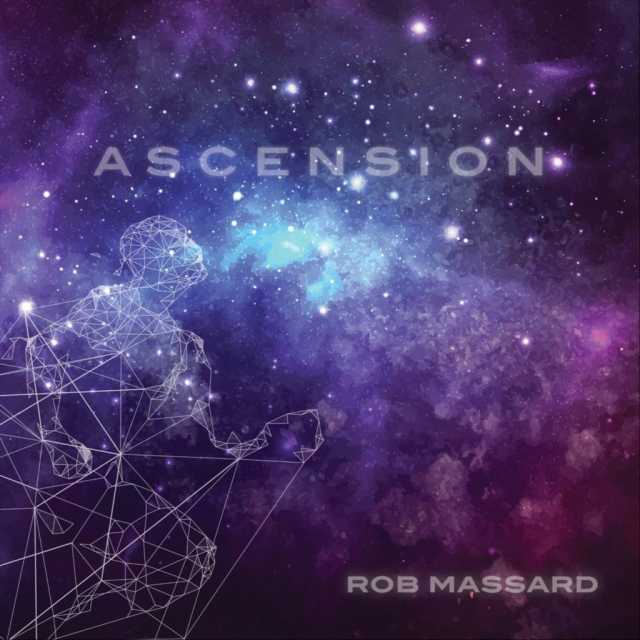Art, History, Music, Politics, and Spirituality For The Modern Alchemist – circulation in over 129 countries

# The Sign of Yuvho Year 18,002 Begins November 10th 2014

There are eight months in the Calendar of Mu, sometimes referred to as the Nyarzirian Calendar. Each month consists of forty-five days, and represents a system of the eight hexagrams moving through the five elements. The Ninzuwu Priest/Priestess invokes the mudra/mantra formulae associated with each hexagram that rules a particular day after invoking the Armor of Amaterasu Ohkami. The chart below illustrates the daily Vasuh letters invoked and the ruling “deity” for the said hexagram:
1. November 10th 2014 = Iut = 16th Hexagram = Aum-Hmu-Hmu
2. November 11th 2014 = Aeuq = 32nd Hexagram = Tuu-Aum
3. November 12th 2014 = Jimmu = 34th Hexagram = Tuu-Lewhu
4. November 13th 2014 = Wosado = 40th Hexagram = Aum-Tuu-Tuu- Phe-Bnhu
5. November 14th 2014 = Yuvho = 51st Hexagram = Zhee-Tuu-Aum-Shki
6. November 15th 2014 = Viyah = 54th Hexagram = Zhee-Lewhu-Aum-Zhee-Zhee
7. November 16th 2014 = Malukedek = 55th Hexagram = Aum-Lewhu
8. November 17th 2014 = Raijin = 62 Hexagram = Phe-Aum
9. November 18th 2014 == Entering Earth Element
10. November 19th 2014 = Iut = 16th Hexagram = Aum-Hmu-Hmu
11. November 20th 2014 = Aeuq = 32nd Hexagram = Tuu-Aum
12. November 21th 2014 = Jimmu = 34th Hexagram = Tuu-Lewhu
13. November 22th 2014 = Wosado = 40th Hexagram = Aum-Tuu-Tuu- Phe-Bnhu
14. November 23th 2014 = Yuvho = 51st Hexagram = Zhee-Tuu-Aum-Shki
15. November 24th 2014 = Viyah = 54th Hexagram = Zhee-Lewhu-Aum-Zhee-Zhee
16. November 25th 2014 = Malukedek = 55th Hexagram = Aum-Lewhu
17. November 26th 2014 = Raijin = 62 Hexagram = Phe-Aum
18. November 27th 2014 == Entering Water Element
19. November 28th 2014 = Iut = 16th Hexagram = Aum-Hmu-Hmu
20. November 29th 2014 = Aeuq = 32nd Hexagram = Tuu-Aum
21. November 30th 2014 = Jimmu = 34th Hexagram = Tuu-Lewhu
22. December 1st 2014 = Wosado = 40th Hexagram = Aum-Tuu-Tuu- Phe-Bnhu
23. December 2nd 2014 = Yuvho = 51st Hexagram = Zhee-Tuu-Aum-Shki
24. December 3rd 2014 = Viyah = 54th Hexagram = Zhee-Lewhu-Aum-Zhee-Zhee
25. December 4th 2014 = Malukedek = 55th Hexagram = Aum-Lewhu
26. December 5th 2014 = Raijin = 62 Hexagram = Phe-Aum
27. December 6th 2014 == Entering Fire Element
28. December 7th 2014 = Iut = 16th Hexagram = Aum-Hmu-Hmu
29. December 8th 2014 = Aeuq = 32nd Hexagram = Tuu-Aum
30. December 9th 2014 = Jimmu = 34th Hexagram = Tuu-Lewhu
31. December 10th 2014 = Wosado = 40th Hexagram = Aum-Tuu-Tuu- Phe-Bnhu
32. December 11th 2014 = Yuvho = 51st Hexagram = Zhee-Tuu-Aum-Shki
33. December 12th 2014 = Viyah = 54th Hexagram = Zhee-Lewhu-Aum-Zhee-Zhee
34. December 13th 2014 = Malukedek = 55th Hexagram = Aum-Lewhu
35. December 14th 2014 = Raijin = 62 Hexagram = Phe-Aum
36. December 15th 2014 == Entering Air Element
37. December 16th 2014 = Iut = 16th Hexagram = Aum-Hmu-Hmu
38. December 17th 2014 = Aeuq = 32nd Hexagram = Tuu-Aum
39. December 18th 2014 = Jimmu = 34th Hexagram = Tuu-Lewhu
40. December 19th 2014 = Wosado = 40th Hexagram = Aum-Tuu-Tuu- Phe-Bnhu
41. December 20th 2014 = Yuvho = 51st Hexagram = Zhee-Tuu-Aum-Shki
42. December 21th 2014 = Viyah = 54th Hexagram = Zhee-Lewhu-Aum-Zhee-Zhee
43. December 22nd 2014 = Malukedek = 55th Hexagram = Aum-Lewhu
44. December 23rd 2014 = Raijin = 62 Hexagram = Phe-Aum
45. December 24th 2014 == Entering Void Element

#### You may have missed#### October by Mae Simpson#### Night Owl by Adrian John Szozda